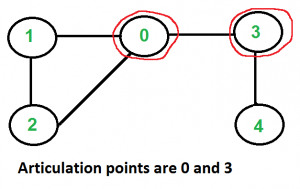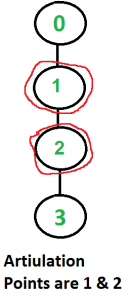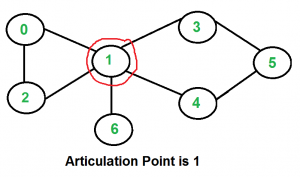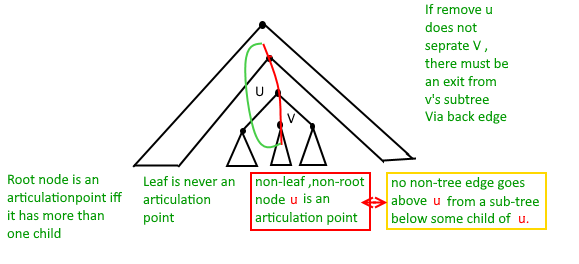GeeksforGeeks App
Open AppBrowser
Continue

# Articulation Points (or Cut Vertices) in a Graph

Given a graph, the task is to find the articulation points in the given graph.

Note: A vertex in an undirected connected graph is an articulation point (or cut vertex) if removing it (and edges through it) disconnects the graph. Articulation points represent vulnerabilities in a connected network – single points whose failure would split the network into 2 or more components. They are useful for designing reliable networks. For a disconnected undirected graph, an articulation point is a vertex removal which increases the number of connected components.

Examples:

Input:Output: 0, 3

Input:Output: 1, 2

Input:Output: 1

Naive Approach:

Below is the idea to solve the problem:

A simple approach is to one by one remove all vertices and see if removal of a vertex causes disconnected graph.

Following the below steps to Implement the idea:

• Iterate over all the vertices and for every vertex do the following:
• Remove v from graph
• See if the graph remains connected (We can either use BFS or DFS)
• Add v back to the graph

Time Complexity: O(V*(V+E)) for a graph represented using an adjacency list.
Auxiliary Space: O(V+E)

## Articulation Points (or Cut Vertices) in a Graph using Tarjan’s Algorithm:

The idea is to use DFS (Depth First Search). In DFS, follow vertices in a tree form called the DFS tree. In the DFS tree, a vertex u is the parent of another vertex v, if v is discovered by u

In DFS tree, a vertex u is an articulation point if one of the following two conditions is true.

• u is the root of the DFS tree and it has at least two children.
• u is not the root of the DFS tree and it has a child v such that no vertex in the subtree rooted with v has a back edge to one of the ancestors in DFS tree of u.

The following figure shows the same points as above with one additional point that a leaf in DFS Tree can never be an articulation point.Follow the below steps to Implement the idea:

• Do DFS traversal of the given graph
• In DFS traversal, maintain a parent[] array where parent[u] stores the parent of vertex u.
• To check if u is the root of the DFS tree and it has at least two children. For every vertex, count children. If the currently visited vertex u is root (parent[u] is NULL) and has more than two children, print it.
• To handle a second case where u is not the root of the DFS tree and it has a child v such that no vertex in the subtree rooted with v has a back edge to one of the ancestors in DFS tree of u maintain an array disc[] to store the discovery time of vertices.
• For every node u, find out the earliest visited vertex (the vertex with minimum discovery time) that can be reached from the subtree rooted with u. So we maintain an additional array low[] such that:
low[u] = min(disc[u], disc[w]) , Here w is an ancestor of u and there is a back edge from some descendant of u to w.

Below is the Implementation of the above approach:

## C++

 `// C++ program to find articulation points in an undirected graph``#include ``using` `namespace` `std;` `// A recursive function that find articulation``// points using DFS traversal``// adj[] --> Adjacency List representation of the graph``// u --> The vertex to be visited next``// visited[] --> keeps track of visited vertices``// disc[] --> Stores discovery times of visited vertices``// low[] -- >> earliest visited vertex (the vertex with minimum``// discovery time) that can be reached from subtree``// rooted with current vertex``// parent --> Stores the parent vertex in DFS tree``// isAP[] --> Stores articulation points``void` `APUtil(vector<``int``> adj[], ``int` `u, ``bool` `visited[],``            ``int` `disc[], ``int` `low[], ``int``& ``time``, ``int` `parent,``            ``bool` `isAP[])``{``    ``// Count of children in DFS Tree``    ``int` `children = 0;` `    ``// Mark the current node as visited``    ``visited[u] = ``true``;` `    ``// Initialize discovery time and low value``    ``disc[u] = low[u] = ++``time``;` `    ``// Go through all vertices adjacent to this``    ``for` `(``auto` `v : adj[u]) {``        ``// If v is not visited yet, then make it a child of u``        ``// in DFS tree and recur for it``        ``if` `(!visited[v]) {``            ``children++;``            ``APUtil(adj, v, visited, disc, low, ``time``, u, isAP);` `            ``// Check if the subtree rooted with v has``            ``// a connection to one of the ancestors of u``            ``low[u] = min(low[u], low[v]);` `            ``// If u is not root and low value of one of``            ``// its child is more than discovery value of u.``            ``if` `(parent != -1 && low[v] >= disc[u])``                ``isAP[u] = ``true``;``        ``}` `        ``// Update low value of u for parent function calls.``        ``else` `if` `(v != parent)``            ``low[u] = min(low[u], disc[v]);``    ``}` `    ``// If u is root of DFS tree and has two or more children.``    ``if` `(parent == -1 && children > 1)``        ``isAP[u] = ``true``;``}` `void` `AP(vector<``int``> adj[], ``int` `V)``{``    ``int` `disc[V] = { 0 };``    ``int` `low[V];``    ``bool` `visited[V] = { ``false` `};``    ``bool` `isAP[V] = { ``false` `};``    ``int` `time` `= 0, par = -1;` `    ``// Adding this loop so that the``    ``// code works even if we are given``    ``// disconnected graph``    ``for` `(``int` `u = 0; u < V; u++)``        ``if` `(!visited[u])``            ``APUtil(adj, u, visited, disc, low,``                   ``time``, par, isAP);` `    ``// Printing the APs``    ``for` `(``int` `u = 0; u < V; u++)``        ``if` `(isAP[u] == ``true``)``            ``cout << u << ``" "``;``}` `// Utility function to add an edge``void` `addEdge(vector<``int``> adj[], ``int` `u, ``int` `v)``{``    ``adj[u].push_back(v);``    ``adj[v].push_back(u);``}` `int` `main()``{``    ``// Create graphs given in above diagrams``    ``cout << ``"Articulation points in first graph \n"``;``    ``int` `V = 5;``    ``vector<``int``> adj1[V];``    ``addEdge(adj1, 1, 0);``    ``addEdge(adj1, 0, 2);``    ``addEdge(adj1, 2, 1);``    ``addEdge(adj1, 0, 3);``    ``addEdge(adj1, 3, 4);``    ``AP(adj1, V);` `    ``cout << ``"\nArticulation points in second graph \n"``;``    ``V = 4;``    ``vector<``int``> adj2[V];``    ``addEdge(adj2, 0, 1);``    ``addEdge(adj2, 1, 2);``    ``addEdge(adj2, 2, 3);``    ``AP(adj2, V);` `    ``cout << ``"\nArticulation points in third graph \n"``;``    ``V = 7;``    ``vector<``int``> adj3[V];``    ``addEdge(adj3, 0, 1);``    ``addEdge(adj3, 1, 2);``    ``addEdge(adj3, 2, 0);``    ``addEdge(adj3, 1, 3);``    ``addEdge(adj3, 1, 4);``    ``addEdge(adj3, 1, 6);``    ``addEdge(adj3, 3, 5);``    ``addEdge(adj3, 4, 5);``    ``AP(adj3, V);` `    ``return` `0;``}`

## Java

 `// A Java program to find articulation``// points in an undirected graph``import` `java.util.*;` `class` `Graph {` `    ``static` `int` `time;` `    ``static` `void` `addEdge(ArrayList > adj, ``int` `u, ``int` `v)``    ``{``        ``adj.get(u).add(v);``        ``adj.get(v).add(u);``    ``}` `    ``static` `void` `APUtil(ArrayList > adj, ``int` `u,``                       ``boolean` `visited[], ``int` `disc[], ``int` `low[],``                       ``int` `parent, ``boolean` `isAP[])``    ``{``        ``// Count of children in DFS Tree``        ``int` `children = ``0``;` `        ``// Mark the current node as visited``        ``visited[u] = ``true``;` `        ``// Initialize discovery time and low value``        ``disc[u] = low[u] = ++time;` `        ``// Go through all vertices adjacent to this``        ``for` `(Integer v : adj.get(u)) {``            ``// If v is not visited yet, then make it a child of u``            ``// in DFS tree and recur for it``            ``if` `(!visited[v]) {``                ``children++;``                ``APUtil(adj, v, visited, disc, low, u, isAP);` `                ``// Check if the subtree rooted with v has``                ``// a connection to one of the ancestors of u``                ``low[u] = Math.min(low[u], low[v]);` `                ``// If u is not root and low value of one of``                ``// its child is more than discovery value of u.``                ``if` `(parent != -``1` `&& low[v] >= disc[u])``                    ``isAP[u] = ``true``;``            ``}` `            ``// Update low value of u for parent function calls.``            ``else` `if` `(v != parent)``                ``low[u] = Math.min(low[u], disc[v]);``        ``}` `        ``// If u is root of DFS tree and has two or more children.``        ``if` `(parent == -``1` `&& children > ``1``)``            ``isAP[u] = ``true``;``    ``}` `    ``static` `void` `AP(ArrayList > adj, ``int` `V)``    ``{``        ``boolean``[] visited = ``new` `boolean``[V];``        ``int``[] disc = ``new` `int``[V];``        ``int``[] low = ``new` `int``[V];``        ``boolean``[] isAP = ``new` `boolean``[V];``        ``int` `time = ``0``, par = -``1``;` `        ``// Adding this loop so that the``        ``// code works even if we are given``        ``// disconnected graph``        ``for` `(``int` `u = ``0``; u < V; u++)``            ``if` `(visited[u] == ``false``)``                ``APUtil(adj, u, visited, disc, low, par, isAP);` `        ``for` `(``int` `u = ``0``; u < V; u++)``            ``if` `(isAP[u] == ``true``)``                ``System.out.print(u + ``" "``);``        ``System.out.println();``    ``}` `    ``public` `static` `void` `main(String[] args)``    ``{` `        ``// Creating first example graph``        ``int` `V = ``5``;``        ``ArrayList > adj1 =``                         ``new` `ArrayList >(V);``        ``for` `(``int` `i = ``0``; i < V; i++)``            ``adj1.add(``new` `ArrayList());``        ``addEdge(adj1, ``1``, ``0``);``        ``addEdge(adj1, ``0``, ``2``);``        ``addEdge(adj1, ``2``, ``1``);``        ``addEdge(adj1, ``0``, ``3``);``        ``addEdge(adj1, ``3``, ``4``);``        ``System.out.println(``"Articulation points in first graph"``);``        ``AP(adj1, V);` `        ``// Creating second example graph``        ``V = ``4``;``        ``ArrayList > adj2 =``                         ``new` `ArrayList >(V);``        ``for` `(``int` `i = ``0``; i < V; i++)``            ``adj2.add(``new` `ArrayList());` `        ``addEdge(adj2, ``0``, ``1``);``        ``addEdge(adj2, ``1``, ``2``);``        ``addEdge(adj2, ``2``, ``3``);` `        ``System.out.println(``"Articulation points in second graph"``);``        ``AP(adj2, V);` `        ``// Creating third example graph``        ``V = ``7``;``        ``ArrayList > adj3 =``                            ``new` `ArrayList >(V);``        ``for` `(``int` `i = ``0``; i < V; i++)``            ``adj3.add(``new` `ArrayList());` `        ``addEdge(adj3, ``0``, ``1``);``        ``addEdge(adj3, ``1``, ``2``);``        ``addEdge(adj3, ``2``, ``0``);``        ``addEdge(adj3, ``1``, ``3``);``        ``addEdge(adj3, ``1``, ``4``);``        ``addEdge(adj3, ``1``, ``6``);``        ``addEdge(adj3, ``3``, ``5``);``        ``addEdge(adj3, ``4``, ``5``);` `        ``System.out.println(``"Articulation points in third graph"``);` `        ``AP(adj3, V);``    ``}``}`

## Python3

 `# Python program to find articulation points in an undirected graph`` ` `from` `collections ``import` `defaultdict`` ` `# This class represents an undirected graph``# using adjacency list representation``class` `Graph:`` ` `    ``def` `__init__(``self``, vertices):``        ``self``.V ``=` `vertices ``# No. of vertices``        ``self``.graph ``=` `defaultdict(``list``) ``# default dictionary to store graph``        ``self``.Time ``=` `0`` ` `    ``# function to add an edge to graph``    ``def` `addEdge(``self``, u, v):``        ``self``.graph[u].append(v)``        ``self``.graph[v].append(u)`` ` `    ``'''A recursive function that find articulation points``    ``using DFS traversal``    ``u --> The vertex to be visited next``    ``visited[] --> keeps track of visited vertices``    ``disc[] --> Stores discovery times of visited vertices``    ``parent[] --> Stores parent vertices in DFS tree``    ``ap[] --> Store articulation points'''``    ``def` `APUtil(``self``, u, visited, ap, parent, low, disc):` `        ``# Count of children in current node``        ``children ``=` `0` `        ``# Mark the current node as visited and print it``        ``visited[u]``=` `True` `        ``# Initialize discovery time and low value``        ``disc[u] ``=` `self``.Time``        ``low[u] ``=` `self``.Time``        ``self``.Time ``+``=` `1` `        ``# Recur for all the vertices adjacent to this vertex``        ``for` `v ``in` `self``.graph[u]:``            ``# If v is not visited yet, then make it a child of u``            ``# in DFS tree and recur for it``            ``if` `visited[v] ``=``=` `False` `:``                ``parent[v] ``=` `u``                ``children ``+``=` `1``                ``self``.APUtil(v, visited, ap, parent, low, disc)` `                ``# Check if the subtree rooted with v has a connection to``                ``# one of the ancestors of u``                ``low[u] ``=` `min``(low[u], low[v])` `                ``# u is an articulation point in following cases``                ``# (1) u is root of DFS tree and has two or more children.``                ``if` `parent[u] ``=``=` `-``1` `and` `children > ``1``:``                    ``ap[u] ``=` `True` `                ``#(2) If u is not root and low value of one of its child is more``                ``# than discovery value of u.``                ``if` `parent[u] !``=` `-``1` `and` `low[v] >``=` `disc[u]:``                    ``ap[u] ``=` `True`   `                    ` `                ``# Update low value of u for parent function calls   ``            ``elif` `v !``=` `parent[u]:``                ``low[u] ``=` `min``(low[u], disc[v])`  `    ``# The function to do DFS traversal. It uses recursive APUtil()``    ``def` `AP(``self``):`` ` `        ``# Mark all the vertices as not visited``        ``# and Initialize parent and visited,``        ``# and ap(articulation point) arrays``        ``visited ``=` `[``False``] ``*` `(``self``.V)``        ``disc ``=` `[``float``(``"Inf"``)] ``*` `(``self``.V)``        ``low ``=` `[``float``(``"Inf"``)] ``*` `(``self``.V)``        ``parent ``=` `[``-``1``] ``*` `(``self``.V)``        ``ap ``=` `[``False``] ``*` `(``self``.V) ``# To store articulation points` `        ``# Call the recursive helper function``        ``# to find articulation points``        ``# in DFS tree rooted with vertex 'i'``        ``for` `i ``in` `range``(``self``.V):``            ``if` `visited[i] ``=``=` `False``:``                ``self``.APUtil(i, visited, ap, parent, low, disc)` `        ``for` `index, value ``in` `enumerate` `(ap):``            ``if` `value ``=``=` `True``: ``print` `(index,end``=``" "``)` ` ``# Create a graph given in the above diagram``g1 ``=` `Graph(``5``)``g1.addEdge(``1``, ``0``)``g1.addEdge(``0``, ``2``)``g1.addEdge(``2``, ``1``)``g1.addEdge(``0``, ``3``)``g1.addEdge(``3``, ``4``)`` ` `print` `(``"\nArticulation points in first graph "``)``g1.AP()` `g2 ``=` `Graph(``4``)``g2.addEdge(``0``, ``1``)``g2.addEdge(``1``, ``2``)``g2.addEdge(``2``, ``3``)``print` `(``"\nArticulation points in second graph "``)``g2.AP()` ` ` `g3 ``=` `Graph (``7``)``g3.addEdge(``0``, ``1``)``g3.addEdge(``1``, ``2``)``g3.addEdge(``2``, ``0``)``g3.addEdge(``1``, ``3``)``g3.addEdge(``1``, ``4``)``g3.addEdge(``1``, ``6``)``g3.addEdge(``3``, ``5``)``g3.addEdge(``4``, ``5``)``print` `(``"\nArticulation points in third graph "``)``g3.AP()` `# This code is contributed by Neelam Yadav`

## C#

 `// A C# program to find articulation``// points in an undirected graph``using` `System;``using` `System.Collections.Generic;` `// This class represents an undirected graph``// using adjacency list representation``public` `class` `Graph {``    ``private` `int` `V; ``// No. of vertices` `    ``// Array of lists for Adjacency List Representation``    ``private` `List<``int``>[] adj;``    ``int` `time = 0;``    ``static` `readonly` `int` `NIL = -1;` `    ``// Constructor``    ``Graph(``int` `v)``    ``{``        ``V = v;``        ``adj = ``new` `List<``int``>[v];``        ``for` `(``int` `i = 0; i < v; ++i)``            ``adj[i] = ``new` `List<``int``>();``    ``}` `    ``// Function to add an edge into the graph``    ``void` `addEdge(``int` `v, ``int` `w)``    ``{``        ``adj[v].Add(w); ``// Add w to v's list.``        ``adj[w].Add(v); ``// Add v to w's list``    ``}` `    ``// A recursive function that find articulation points using DFS``    ``// u --> The vertex to be visited next``    ``// visited[] --> keeps track of visited vertices``    ``// disc[] --> Stores discovery times of visited vertices``    ``// parent[] --> Stores parent vertices in DFS tree``    ``// ap[] --> Store articulation points``    ``void` `APUtil(``int` `u, ``bool``[] visited, ``int``[] disc,``                ``int``[] low, ``int``[] parent, ``bool``[] ap)``    ``{` `        ``// Count of children in DFS Tree``        ``int` `children = 0;` `        ``// Mark the current node as visited``        ``visited[u] = ``true``;` `        ``// Initialize discovery time and low value``        ``disc[u] = low[u] = ++time;` `        ``// Go through all vertices adjacent to this``        ``foreach``(``int` `i ``in` `adj[u])``        ``{``            ``int` `v = i; ``// v is current adjacent of u` `            ``// If v is not visited yet, then make it a child of u``            ``// in DFS tree and recur for it``            ``if` `(!visited[v]) {``                ``children++;``                ``parent[v] = u;``                ``APUtil(v, visited, disc, low, parent, ap);` `                ``// Check if the subtree rooted with v has``                ``// a connection to one of the ancestors of u``                ``low[u] = Math.Min(low[u], low[v]);` `                ``// u is an articulation point in following cases` `                ``// (1) u is root of DFS tree and has two or more children.``                ``if` `(parent[u] == NIL && children > 1)``                    ``ap[u] = ``true``;` `                ``// (2) If u is not root and low value of one of its child``                ``// is more than discovery value of u.``                ``if` `(parent[u] != NIL && low[v] >= disc[u])``                    ``ap[u] = ``true``;``            ``}` `            ``// Update low value of u for parent function calls.``            ``else` `if` `(v != parent[u])``                ``low[u] = Math.Min(low[u], disc[v]);``        ``}``    ``}` `    ``// The function to do DFS traversal.``    ``// It uses recursive function APUtil()``    ``void` `AP()``    ``{``        ``// Mark all the vertices as not visited``        ``bool``[] visited = ``new` `bool``[V];``        ``int``[] disc = ``new` `int``[V];``        ``int``[] low = ``new` `int``[V];``        ``int``[] parent = ``new` `int``[V];``        ``bool``[] ap = ``new` `bool``[V]; ``// To store articulation points` `        ``// Initialize parent and visited, and``        ``// ap(articulation point) arrays``        ``for` `(``int` `i = 0; i < V; i++) {``            ``parent[i] = NIL;``            ``visited[i] = ``false``;``            ``ap[i] = ``false``;``        ``}` `        ``// Call the recursive helper function to find articulation``        ``// points in DFS tree rooted with vertex 'i'``        ``for` `(``int` `i = 0; i < V; i++)``            ``if` `(visited[i] == ``false``)``                ``APUtil(i, visited, disc, low, parent, ap);` `        ``// Now ap[] contains articulation points, print them``        ``for` `(``int` `i = 0; i < V; i++)``            ``if` `(ap[i] == ``true``)``                ``Console.Write(i + ``" "``);``    ``}` `    ``// Driver method``    ``public` `static` `void` `Main(String[] args)``    ``{``        ``// Create graphs given in above diagrams``        ``Console.WriteLine(``"Articulation points in first graph "``);``        ``Graph g1 = ``new` `Graph(5);``        ``g1.addEdge(1, 0);``        ``g1.addEdge(0, 2);``        ``g1.addEdge(2, 1);``        ``g1.addEdge(0, 3);``        ``g1.addEdge(3, 4);``        ``g1.AP();``        ``Console.WriteLine();` `        ``Console.WriteLine(``"Articulation points in Second graph"``);``        ``Graph g2 = ``new` `Graph(4);``        ``g2.addEdge(0, 1);``        ``g2.addEdge(1, 2);``        ``g2.addEdge(2, 3);``        ``g2.AP();``        ``Console.WriteLine();` `        ``Console.WriteLine(``"Articulation points in Third graph "``);``        ``Graph g3 = ``new` `Graph(7);``        ``g3.addEdge(0, 1);``        ``g3.addEdge(1, 2);``        ``g3.addEdge(2, 0);``        ``g3.addEdge(1, 3);``        ``g3.addEdge(1, 4);``        ``g3.addEdge(1, 6);``        ``g3.addEdge(3, 5);``        ``g3.addEdge(4, 5);``        ``g3.AP();``    ``}``}` `// This code is contributed by PrinciRaj1992`

## Javascript

 ``

Output

```Articulation points in first graph
0 3
Articulation points in second graph
1 2
Articulation points in third graph
1 ```

Time Complexity: O(V+E), For DFS it takes O(V+E) time.
Auxiliary Space: O(V+E), For visited array, adjacency list array.

My Personal Notes arrow_drop_up